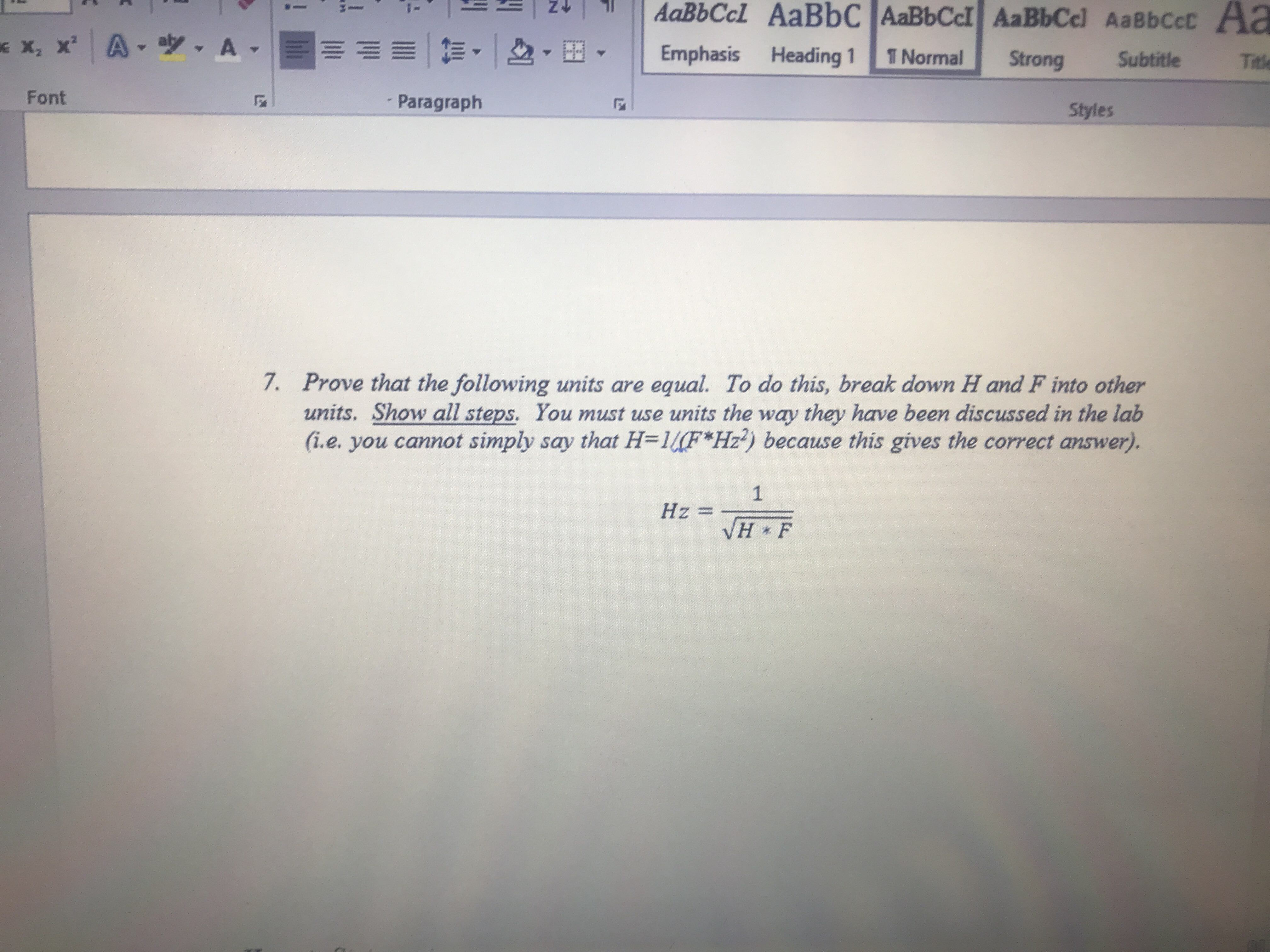着一i-x2 x' | A ▼ツ. A-11! t= ▼ | D2 ▼田| | Emphasis Heading i | Normal 1 StrongSubtitleTitleFontParagraphStyles7.Prove that the following units are equal. To do this, break down H and F into otherunits. Show all steps, You must use units the way they have been discussed in the lab(ie, you cannot simply say that H=14F*Hz) because this gives the correct answer.Hz =H * F

Questionhelp_outlineImage Transcriptionclose着一 i- x2 x' | A ▼ツ. A-11 ! t= ▼ | D2 ▼田 | | Emphasis Heading i | Normal 1 Strong Subtitle Title Font Paragraph Styles 7. Prove that the following units are equal. To do this, break down H and F into other units. Show all steps, You must use units the way they have been discussed in the lab (ie, you cannot simply say that H=14F*Hz) because this gives the correct answer. Hz = H * F fullscreen
Step 1

The unit of frequency is hertz (Hz). The unit of inductance is henry (H). The unit of capacitance is farad (F). The unit of charge is coulomb (C). The unit of voltage is volt (V). The unit of time is second (s). The unit of current is ampere (A).

Step 2

Simplify the right hand si...

Want to see the full answer?

See Solution

Want to see this answer and more?

Our solutions are written by experts, many with advanced degrees, and available 24/7

See Solution
Tagged in

Units and Dimensions